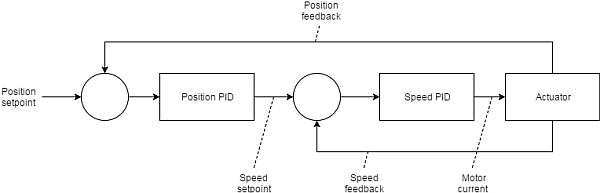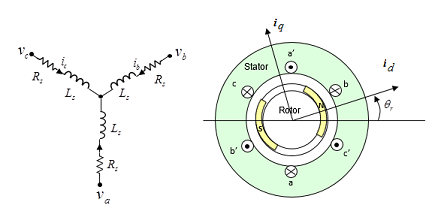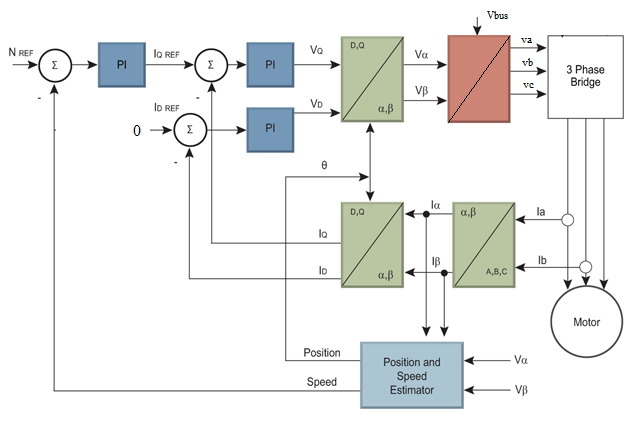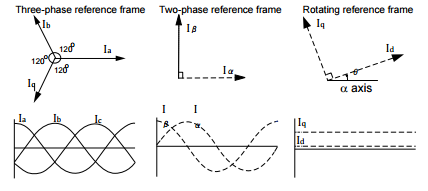# cascaded position and speed motor controller simulation (for an FOC controlled motor)24 0.5 0.1 1800 100

About: This is a Javascript online simulation of cascaded position and speed motor control for an FOC controlled motor. Use sliders to set params.

### Controller model```Position loop:
pos_error = pos_setpoint - pos_feedback
pos_integral += pos_error * pos_integrator_gain
pos_differential = delta(pos_error) * pos_differential_gain
vel_cmd = pos_error * pos_gain + pos_integral + pos_differential
```
```Velocity loop:
vel_error = vel_cmd - vel_feedback
vel_integral += vel_error * vel_integrator_gain
vel_differential = delta(vel_error) * vel_differential_gain
current_cmd = vel_error * vel_gain + vel_integral + vel_differential
```

### Motor model```Motor model:
Kt = 0.2 	// Torque constant [Nm/A]
Kv = 0.2	// Motor velocity constant or back EMF constant [V/(rad/s)]
R = 2.5 	// Phase Resistance [Ohm]
L = 2.5e-3 	// Phase Inductance [H]
J = 1e-3  	// Rotor Inertia [kg·m^2]
B = 1.0e-4	// Rotor Friction [Nm/(rad/s)]

tm = 62.5e-3	// mechanical time constant tm = R·J/(Kt·Kv) [s]
te = 1e-3	// electrical time constant te = L/R [s]

Motor equations:
v = R·i + L·di/dt + ve
ve = Kv·ω

Te = Kt·i
Te = TL + B·ω + JL·dω/dt;

where:
v - voltage [V]
i - current [A]
ve - back electromotive force [V]
JL - Load inertia + Rotor inertia [kg·m^2]
```

### FOC control algorithm```Clarke transformation into orthogonal stacionary reference frame:
iα = ia
iβ = 1/√3·(ia + 2ib);

Park transformation into rotating reference frame
id =  iα·cos(θ) + iβ·sin(θ)
iq = -iα·sin(θ) + iβ·cos(θ)

where:
θ - rotation angle

PID
iq_ref = -PID(rpm_ref-rpm)*Tmax/Kt	// Kp = 5, Ki = 1e-5, Kd = 1000;
Vq = PID(iq_ref-iq);			// Kp = 0.5, Ki=0.01, Kd = 0
Vd = PID(id_ref-id);			// Kp = 0.5, Ki=0.01, Kd = 0

Inverse Park transformation:
Vα =  Vd·cos(θ) - Vq·sin(θ)
Vβ =  Vd·sin(θ) + Vq·cos(θ)

Inverse Clarke transformation
va =          Vα
vb = -sin(30°)*Vα + cos(30°)*Vβ
vc = -sin(30°)*Vα - cos(30°)*Vβ

Voltage offset and amplify
vn = (va+vb+vc)/3;
va = (va-vn)*Vbus
vb = (vn-vn)*Vbus
vc = (vn-vn)*Vbus

```Introduction to FOC controllers: Texas Instruments has a nice introduction into this topic ('Teaching Old Motors New Tricks').